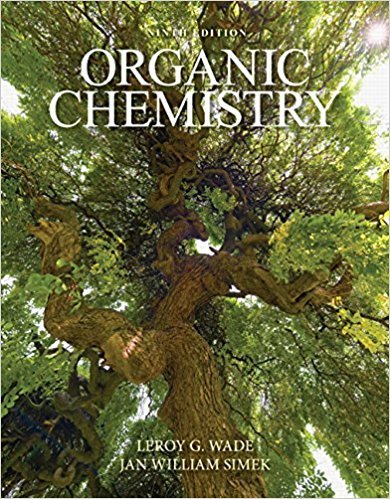×

# Dimethylamine, (CH3)2NH, has a molecular weight of 45 and a boiling point of 7.4 CISBN: 9780321971371 282

## Solution for problem PROBLEM 10-6 Chapter 10

Organic Chemistry | 9th Edition

• Textbook Solutions
• 2901 Step-by-step solutions solved by professors and subject experts
• Get 24/7 help from StudySoup virtual teaching assistantsOrganic Chemistry | 9th Edition

4 5 0 256 Reviews
21
0
Problem PROBLEM 10-6

Dimethylamine, (CH3)2NH, has a molecular weight of 45 and a boiling point of 7.4 C. Trimethylamine, (CH3)3N, has a higher molecular weight (59) but a lower boiling point (3.5 C). Explain this apparent discrepancy.

Step-by-Step Solution:
Step 1 of 3

Chapter 1 2510 IV. (Section 1-4)Lewis Structures A. Rules 1. Draw the desired molecular skeleton CH 4 H H C H H 2. Determine number of valence electrons - - H 4 x 1e = 4e C 1 x 4e = 4e total 8e - 3. Octet rule....

Step 2 of 3

Step 3 of 3

##### ISBN: 9780321971371

The full step-by-step solution to problem: PROBLEM 10-6 from chapter: 10 was answered by , our top Chemistry solution expert on 01/25/18, 04:04PM. Organic Chemistry was written by and is associated to the ISBN: 9780321971371. The answer to “Dimethylamine, (CH3)2NH, has a molecular weight of 45 and a boiling point of 7.4 C. Trimethylamine, (CH3)3N, has a higher molecular weight (59) but a lower boiling point (3.5 C). Explain this apparent discrepancy.” is broken down into a number of easy to follow steps, and 34 words. Since the solution to PROBLEM 10-6 from 10 chapter was answered, more than 372 students have viewed the full step-by-step answer. This textbook survival guide was created for the textbook: Organic Chemistry, edition: 9. This full solution covers the following key subjects: . This expansive textbook survival guide covers 26 chapters, and 1677 solutions.

Unlock Textbook Solution

Dimethylamine, (CH3)2NH, has a molecular weight of 45 and a boiling point of 7.4 C

×